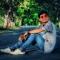Class Central is learner-supported. When you buy through links on our site, we may earn an affiliate commission.

# Engineering Dynamics (Fall 2011)

### Overview

Course Features
• Video lectures
• Captions/transcript
• Lecture notes
• Assignments: problem sets with solutions
• Exams and solutions
• Recitation videos
• Resource Index
Course Description

This course is an introduction to the dynamics and vibrations of lumped-parameter models of mechanical systems. Topics covered include kinematics, force-momentum formulation for systems of particles and rigid bodies in planar motion, work-energy concepts, virtual displacements and virtual work. Students will also become familiar with the following topics: Lagrange's equations for systems of particles and rigid bodies in planar motion, and linearization of equations of motion. After this course, students will be able to evaluate free and forced vibration of linear multi-degree of freedom models of mechanical systems and matrix eigenvalue problems.

### Syllabus

1. History of Dynamics; Motion in Moving Reference Frames.
2. Newton's Laws & Describing the Kinematics of Particles.
3. Motion of Center of Mass; Acceleration in Rotating Ref. Frames.
4. Movement of a Particle in Circular Motion w/ Polar Coordinates.
R2. Velocity and Acceleration in Translating and Rotating Frames.
5. Impulse, Torque, & Angular Momentum for a System of Particles.
6. Torque & the Time Rate of Change of Angular Momentum.
R3. Motion in Moving Reference Frames.
7. Degrees of Freedom, Free Body Diagrams, & Fictitious Forces.
8. Fictitious Forces & Rotating Mass.
R4. Free Body Diagrams.
9. Rotating Imbalance.
10. Equations of Motion, Torque, Angular Momentum of Rigid Bodies.
R5. Equations of Motion.
11. Mass Moment of Inertia of Rigid Bodies.
12. Problem Solving Methods for Rotating Rigid Bodies.
R6. Angular Momentum and Torque.
13. Four Classes of Problems With Rotational Motion.
14. More Complex Rotational Problems & Their Equations of Motion.
R7. Cart and Pendulum, Direct Method.
Notation Systems.
15. Introduction to Lagrange With Examples.
R8. Cart and Pendulum, Lagrange Method.
16. Kinematic Approach to Finding Generalized Forces.
17. Practice Finding EOM Using Lagrange Equations.
R9. Generalized Forces.
18. Quiz Review From Optional Problem Set 8.
19. Introduction to Mechanical Vibration.
20. Linear System Modeling a Single Degree of Freedom Oscillator.
21. Vibration Isolation.
22. Finding Natural Frequencies & Mode Shapes of a 2 DOF System.
23. Vibration by Mode Superposition.
24. Modal Analysis: Orthogonality, Mass Stiffness, Damping Matrix.
R11. Double Pendulum System.
25. Modal Analysis: Response to IC's and to Harmonic Forces.
26. Response of 2-DOF Systems by the Use of Transfer Functions.
27. Vibration of Continuous Structures: Strings, Beams, Rods, etc..
R12. Modal Analysis of a Double Pendulum System.

### Taught by

Prof. J. Kim Vandiver and Prof. David Gossard

## Reviews

5.0 rating, based on 1 Class Central review

Start your review of Engineering Dynamics (Fall 2011)

•YAXHKUMAR PATEL
Wow this is a marvelous course place and learn wary well this course is very beautifull and this is godd to increase my skills

### Never Stop Learning.

Get personalized course recommendations, track subjects and courses with reminders, and more.